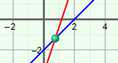# Solving Linear Systems (Standard Form)

### DESCRIPTION

Solve systems of linear equations, written in standard form. Explore what it means to solve systems algebraically (with substitution or elimination) and graphically. Also, use a draggable green point to see what it means when (x, y) values are solutions of an equation, or of a system of equations.

Full Lesson Info